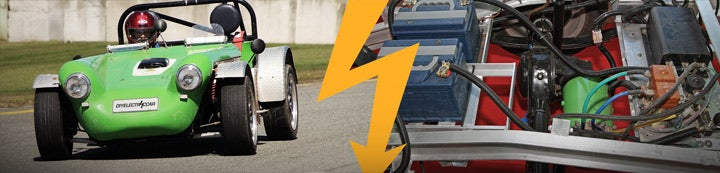1 - 2 of 2 Posts

#### Mastiff

·
##### Registered
Joined
·
369 Posts

For an introduction to Amps, Volts, watts and watt-hours see this wiki article.

Power
If you are familiar with cars, you are probably familiar with the concept of power. Power is what gives the car acceleration and what maintains a car at a given speed. Mechanically power is the product of torque and rpm, so while torque can be multiplied through gearing (by lowering rpm), power is independent of gearing

Now on the electrical side of things power it is important to know that Voltage times Amps = Watts. This is only a measure of instant power, this is the amount of power that flows though an electrical circuit, this does not tell us how long this power can be used. Power is measured in metric units as watts (W) or more commonly kilowatts (1kW=1000W) or horsepower in the imperial units (1hp = 746W). Technically there is no difference between power produced by an ICE or an electric motor, though because the torque curves are so different just comparing the peak power of the two motors isn't sufficient. The peak power of an EV is generally decided by the controller, as long as the batteries and motor can handle that power. For example a Zilla LV 1k can produce 1000 amps at 156V, which is 156kW or ~210hp.

Energy
Energy is what gives an electric car range. It is the product of power and time (E = P x t) or the product of voltage and amp-hours. Energy is stored in the batteries as chemical energy and is converted to kinetic energy by the motor to make the car move. The measurement used for measuring total electrical energy in a battery pack is called Watt-Hours (Wh) or sometimes Kilowatt-Hours (1kWh=1000Wh). Watt-Hours is a measurement we can use to figure out how much energy we have, i.e. how long can we sustain a given level of power.

If a vehicle takes 10kW to travel 55mph, and the owner wishes to do so for two hours then the energy required to do so is power x time or 10 x 2 = 20kWh. So the owner would need to size the battery pack to contain 20kWh in order to travel that distance.

#### rangerdev

·
##### Registered
Joined
·
11 Posts
Thanks for the informative post.

Something I would really like to know is how I can identify a comparable electric motor. Say I have a 5hp briggs sitting in my shop, and a random electric motor sitting beside it that I pulled from something. The EM says 1/2 hp. The briggs is 5hp. How do I know if the EM would be able to keep up with the briggs?

If I have one 12v battery to power this motor, how do I figure out the output power? volts x amps = watts .... watt = 0.00134hp is easy enough but I don't know the amps the battery is putting out. Can I calculate this? Or do I need to measure it?

What I am trying to say is, I hoping for a way that I can look at a motor and say after a few calculations "Yes that will most likely work to replace that ICE or no, that motor is much too small."

I work in an industrial setting and they are constantly tossing out DC motors (because they are too lazy to replace bearings etc.), but I never know what ones would work and which won't. Any advise would be appreciated. Thank you!

1 - 2 of 2 Posts